Previous: 6.2.2 Definition of Wigner Up: 6.2 Quantum Mechanics in Next: 6.2.4 Probabilistic Structure

## 6.2.3 Operator-Theoretic Structure

The Wigner function construction is a special case of a correspondence between quantum-mechanical operators and ordinary c-number phase space functions.

For operators which are functions of coordinates or momenta only, such as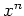or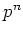, the Weyl transform agrees with the classical function corresponding to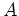. This can not be the case in general, as is clear from the non-commutativity of the operator product. For example the Weyl transform of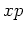is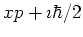.

The crucial question is: what does the operator product look like in phase space? This question was answered by Moyal in [Moy49] by introduction of the star product.

Let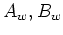be the Weyl transforms of the operators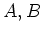. Then the Weyl transform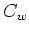of the operator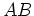is given by the Moyal star product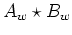:(6.49)

The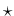-multiplication of c-number phase space functions is in complete isomorphism to Hilbert-space operator multiplication.

The von Neumann equation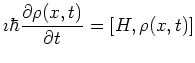(6.50)

becomes the quantum Liouville (or Wigner) equation: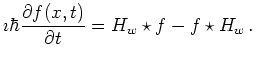(6.51)